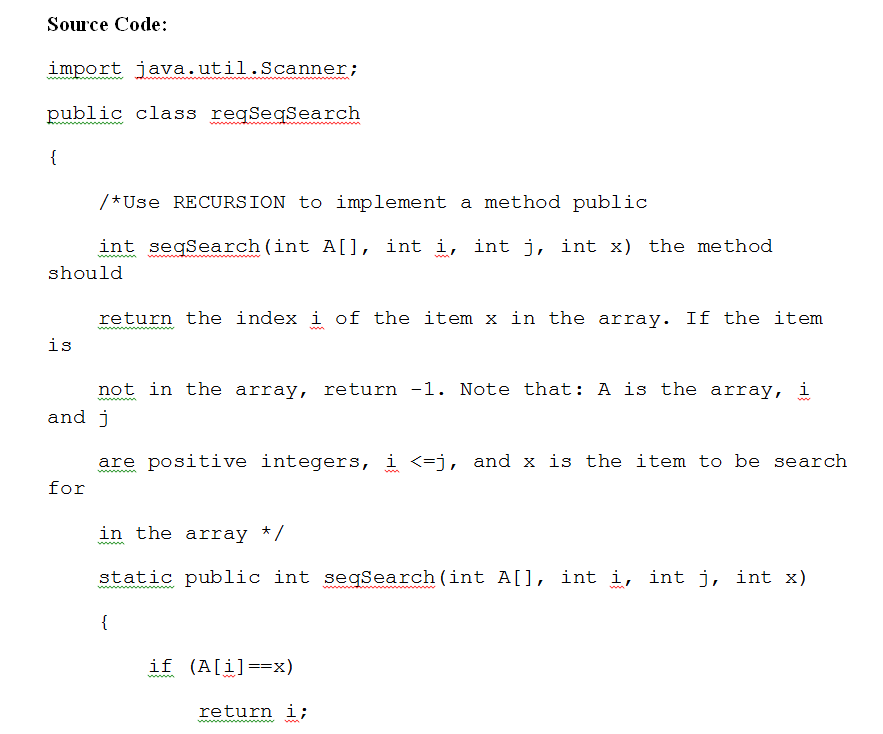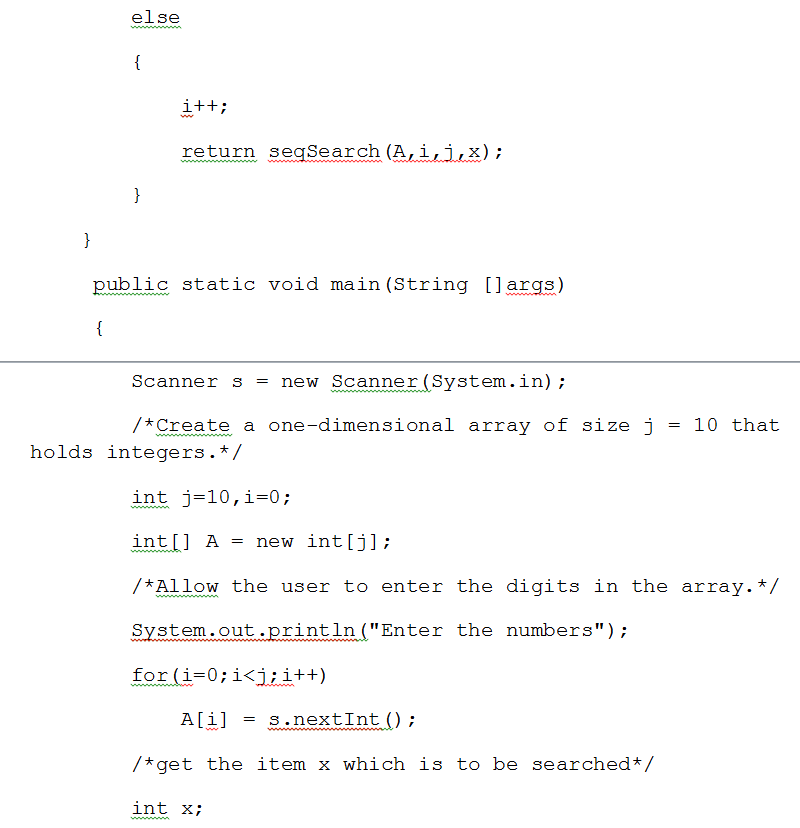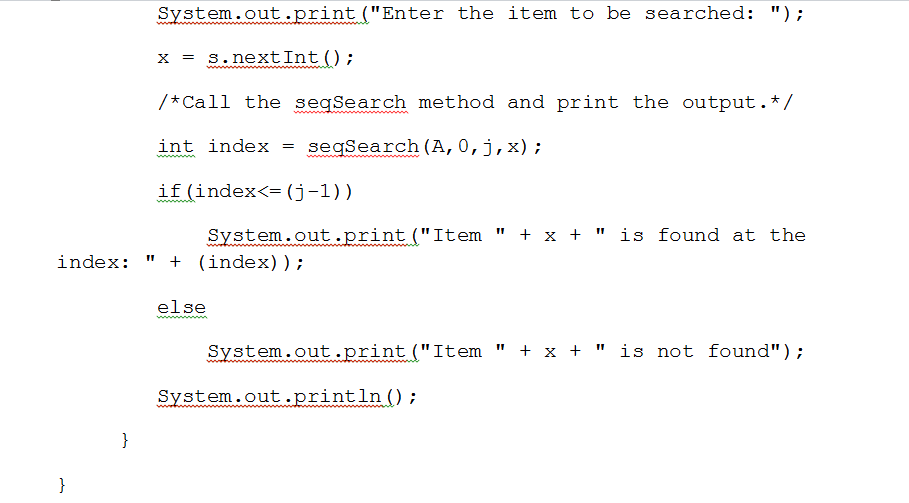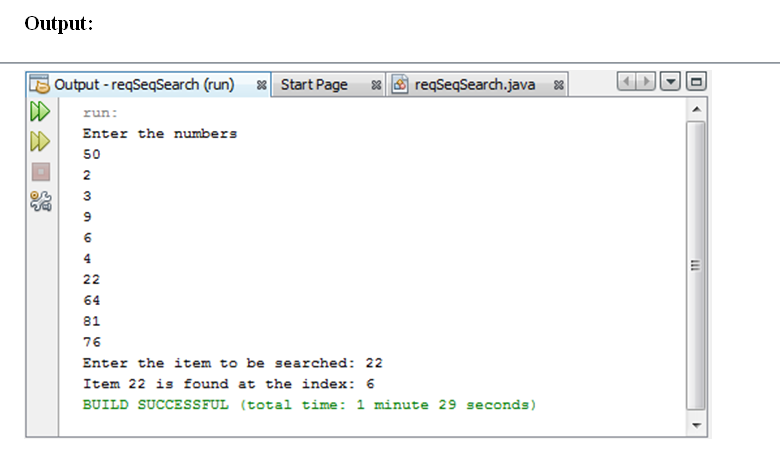# Answered! Create a one-dimensional array of size j = 10 that holds integers. (1 point) B. Allow the user to enter the digits in the array. (1 point)…

Create a one-dimensional array of size j = 10 that holds integers. (1 point) B.

Allow the user to enter the digits in the array. (1 point)

Don't use plagiarized sources. Get Your Custom Essay on
Answered! Create a one-dimensional array of size j = 10 that holds integers. (1 point) B. Allow the user to enter the digits in the array. (1 point)…
GET AN ESSAY WRITTEN FOR YOU FROM AS LOW AS \$13/PAGE

C. Use RECURSION to implement a method public int seqSearch(int A[], int i, int j, int x) the method should return the index i of the item x in the array. If the item is not in the array, return -1. Note that: A is the array, i and j are positive integers, i <=j, and x is the item to be search for in the array A. (3 points)

D. Call your seqSearch method and print the output. (1 point)

(java code)Editable Code:

import java.util.Scanner;
public class reqSeqSearch
{
/*Use RECURSION to implement a method public
int seqSearch(int A[], int i, int j, int x) the method should
return the index i of the item x in the array. If the item is
not in the array, return -1. Note that: A is the array, i and j
are positive integers, i <=j, and x is the item to be search for
in the array */
static public int seqSearch(int A[], int i, int j, int x)
{
if (A[i]==x)
return i;
else
{
i++;
return seqSearch(A,i,j,x);
}
}
public static void main(String []args)
{
Scanner s = new Scanner(System.in);
/*Create a one-dimensional array of size j = 10 that holds integers.*/
int j=10,i=0;
int[] A = new int[j];
/*Allow the user to enter the digits in the array.*/
System.out.println(“Enter the numbers”);
for(i=0;i<j;i++)
A[i] = s.nextInt();
/*get the item x which is to be searched*/
int x;
System.out.print(“Enter the item to be searched: “);
x = s.nextInt();
/*Call the seqSearch method and print the output.*/
int index = seqSearch(A,0,j,x);
if(index<=(j-1))
System.out.print(“Item ” + x + ” is found at the index: ” + (index));
else